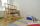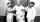Water pool

Pool with volume 990hl completely filled, if water flows by one tap 8 hours and by second tap 6 hours. First tap give 10hl more than second per hour. How many hl flows in each of them in an hour?

Result

a =  75 hl/h
b =  65 hl/h

Solution:

990 = 8 a + 6b
a = 10 + b

8a+6b = 990
a-b = 10

a = 75
b = 65

Calculated by our linear equations calculator.

Leave us a comment of example and its solution (i.e. if it is still somewhat unclear...):Be the first to comment!To solve this example are needed these knowledge from mathematics:

Do you have a linear equation or system of equations and looking for its solution? Or do you have quadratic equation? Tip: Our volume units converter will help you with converion of volume units.

Next similar examples:

1. Two loomsOn two different looms with different performance can be make required quantity of textile in 6 hours when both work together. On the first machine this quantity of the textile can make in 10 hours. How long should take make same quantity of textile on a s
2. Rabbits 3Viju has 40 chickens and rabbits. If in all there are 90 legs. How many rabbits are there with Viju?
3. Football match 4In a football match with the Italy lost 3 goals with Germans. Totally fell 5 goals in the match. Determine the number of goals of Italy and Germany.
4. Jane plantsJane plants flowers in the garden. If she planted 12 every hour instead of 9 flowers, she would finish with the job an hour earlier. How many flowers does she plant?
5. NovakNovak needed to dig up three of the same pit in the garden. The first pit dug father alone for 15 hours. His second dig son helped him and it did that in six hours. The third pit dug son himself. How long it took him?
6. Six workersSix workers planned to repair the roof in 5 days. After two days, two workers get sick. How long will the remaining workers complete the roof at the same rate of work?
7. Two numbersWe have two numbers. Their sum is 140. One-fifth of the first number is equal to half the second number. Determine those unknown numbers.
8. PaintersOne painter could paint a school for 7 days. The second one for 6 days. It was necessary to paint the school in two days. Next painter was invited and all worked together. How long would this painter painted the school itself?
9. Three brothersThe three brothers have a total of 42 years. Jan is five years younger than Peter and Peter is 2 years younger than Michael. How many years has each of them?
10. MushroomsEva and Jane collected 114 mushrooms together. Eve found twice as much as Jane. How many mushrooms found each of them?
11. Father and sonWhen I was 11, my father was 35 years old. Today, my father has three times more years than me. How old is she?
12. Six yearsIn six years Jan will be twice as old as he was six years ago. How old is he?
13. Boys and girlsThere are 48 children in the sports club, boys are 10 more than girls. How many girls go to the club?
14. Three figures - numbersThe sum of three numbers, if each is 10% larger than the previous one, is 662. Determine the figures.
15. Fifth of the numberThe fifth of the number is by 24 less than that number. What is the number?
16. TheatroTheatrical performance was attended by 480 spectators. Women were in the audience 40 more than men and children 60 less than half of adult spectators. How many men, women and children attended a theater performance?
17. EquationsSolve following system of equations: 6(x+7)+4(y-5)=12 2(x+y)-3(-2x+4y)=-44